# Wijsman convergence

Jump to: navigation, search

R. Wijsman [a4] introduced a convergence for sequences of proper lower semi-continuous convex functions in. Workers in topologies on hyperspaces found this convergence and the resulting topology quite useful and subsequently a vast body of literature developed on this topic (see [a1], [a2]).

Suppose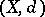is a metric space and let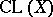denote the family of all non-empty closed subsets of. For eachandone sets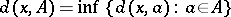. One says that a net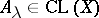(cf. also Net (of sets in a topological space)) is Wijsman convergent to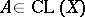if and only if for each,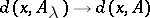, i.e. the convergence is pointwise. The resulting topology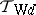onis called the Wijsman topology induced by the metric. The dependence of the Wijsman topology on the metricis quite strong in as much as even two different uniformly equivalent metrics may induce different Wijsman topologies. Necessary and sufficient conditions for two metrics to induce the same Wijsman topology have been found by C. Costantini, S. Levi and J. Zieminska, among others. G. Beer showed that ifis complete and separable (cf. also Complete metric space; Separable space), thenis a Polish space, i.e. it is separable and has a compatible complete metric.

If the pointwise convergenceis replaced by uniform convergence, then Hausdorff convergence is obtained, which has been known for a long time. The associated Hausdorff topologyis derived from the Hausdorff metric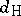given by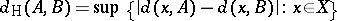). It is known that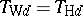if and only ifis totally bounded (cf. also Totally-bounded space).

A natural question arises: What is the supremum of the Wijsman topologies induced by the family of all metrics that are topologically (respectively, uniformly) equivalent to. It was shown by Beer, Levi, A. Lechicki, and S. Naimpally that the supremum of topologically (respectively, uniformly) equivalent metrics is the Vietoris topology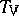(cf. Exponential topology; respectively, the proximal topology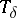). These are hit-and-miss type topologies; the former has been known for a long time while the latter is a rather recent discovery (1999; cf. also Hit-or-miss topology). It is known that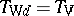if and only ifis compact, while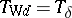is equivalent tobeing totally bounded. G. Di Maio and Naimpally discovered a (hit-and-miss) proximal ball topology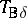which equals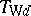in almost convex metric spaces (these include normed linear spaces) [a3]. L. Holá and R. Lucchetti have discovered necessary and sufficient conditions for the equality ofand.

The Wijsman topologyis always a Tikhonov topology (cf. also Tikhonov space) and a remarkable theorem of Levi and Lechicki shows that the separability ofis equivalent tobeing metrizable or first countable or second countable.

Wijsman's original work has been generalized by U. Mosco, Beer and others. Naimpally, Di Maio and Holá have studied Wijsman convergence in function spaces (see [a2]).